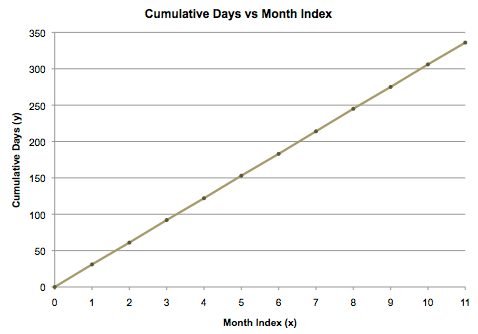< Back

# Calculating the Day of the Year

2012-04-19

## In summary:

Given a date, the day of the year can be calculated:

```var day = Math.floor((367 * month - 362) / 12) + date -
((month > 2) ? (2 - isLeapYear) : 0);
```

Where `month` is 1 - January, 2 - February, etc and `day_of_year` is 1 - 1st Jan, 2 - 2nd Jan, etc

## Introduction

If you are given any date, the number of days since the beginning of the year can be calculated. This value will range between 1 and 366:

DateDay of Year
1 January1
2 January2
31 January31
1 February32
2 February33
28 February59
29 February60 (if day exists)
1 March60 (or 61 for leap years)
31 December365 (or 366 for leap years)

## Linear Equation Approximation

First, we will pretend that February has 30 days, instead of 28 or 29 days. Later we will remove these extra one or two days, if necessary. Now, if we calculate the number of days that have passed in all previous months, which we will call 'cumulative days':

Month NameMonth index (x)Days in MonthCumulative Days (y)
January0310
February130 (for now!)31
March23161
April33092
May431122
June530153
July631183
August731214
September830245
October931275
November1030306
December1131336

Graphing the cumulative days (y) vs. the month index (x):This curve is pretty linear (a straight line), and the slope of the line can be approximated as the total number of days in the year (367, not 365 because we are pretending that February has 30 days) divided by the number of months in the year (12).

367 days / 12 months = 30.58 days/month

The difference between the actual cumulative days (y) and the approximation of 30.58*x can be shown as:

Month Index (x)Cumulative Days (y)30.58 * xy - (30.58 * x)
000+0.00
13130.58+0.42
26161.16−0.16
39291.74+0.26
4122122.32−0.32
5153152.90+0.10
6183183.48−0.48
7214214.06−0.06
8245244.64+0.36
9275275.22−0.22
10306305.80+0.20
11336336.38−0.38

If we round the approximate equation to the nearest integer, the approximate equation then exactly calculates the cumulative days:

```var y = Math.round(30.58 * x);
```

It will be useful later on to use a floor() function instead of a round() function:

```var y = Math.floor(30.58 * x + 0.5);
```

In this equation, x is the Month Index, which has the values (0, 1, 2, …, 11). If we prefer to use conventional month numbers (1, 2, 3, …, 12), we need to shift x by 1:

```var y = Math.floor(30.58 * (month - 1) + 0.5);
```

It is interested to observe that 30.58 is not the only value that will work in this equation. In fact, using any number between 30.5625 and 30.5833 (between 489/16 and 367/12) will result in the correct value.

## Adding the Days for the Current Month

Now we add the number of days in the current month:

```var day = y + date;
```

## Compensating for February

Finally, if the month is March or later we need to remove our extra one or two days to account for February having fewer days:

```if (month > 2)
{
if (isLeapYear) {
day = day - 1;
} else {
day = day - 2;
}
```

This can also be written in ternary form:

```day = day - ((month > 2) ? (2 - isLeapYear) : 0);
```

## Putting It Together

Combined together the expression for the day of the year is:

```day = Math.floor(30.58 * (month - 1) + 0.5) + date -
((month > 2) ? (2 - isLeapYear) : 0);
```

## Using Integer Mathematics

Unlike JavaScript, some programming languages have different data types for integers and floating point variables. It is useful to write the algorithm so that it can work with integer variables and not require floating point calculations:

```var day = Math.floor((367 * month - 362) / 12) + date -
((month > 2) ? (2 - isLeapYear) : 0);
```

## Translating Into Other Languages

It is now trivial to convert this expression into other programming languages. In Perl or PHP, the expression becomes:

```\$day = (367 * \$month - 362) / 12 + \$date -
((\$month > 2) ? (2 - \$isLeapYear) : 0);
```

In the C, C++ or Java programming languages, the expression can be written:

```int day = (367 * month - 362) / 12 + date -
((month > 2) ? (2 - isLeapYear) : 0);
```

## Demo

Year: January February March April May June July August September October November December